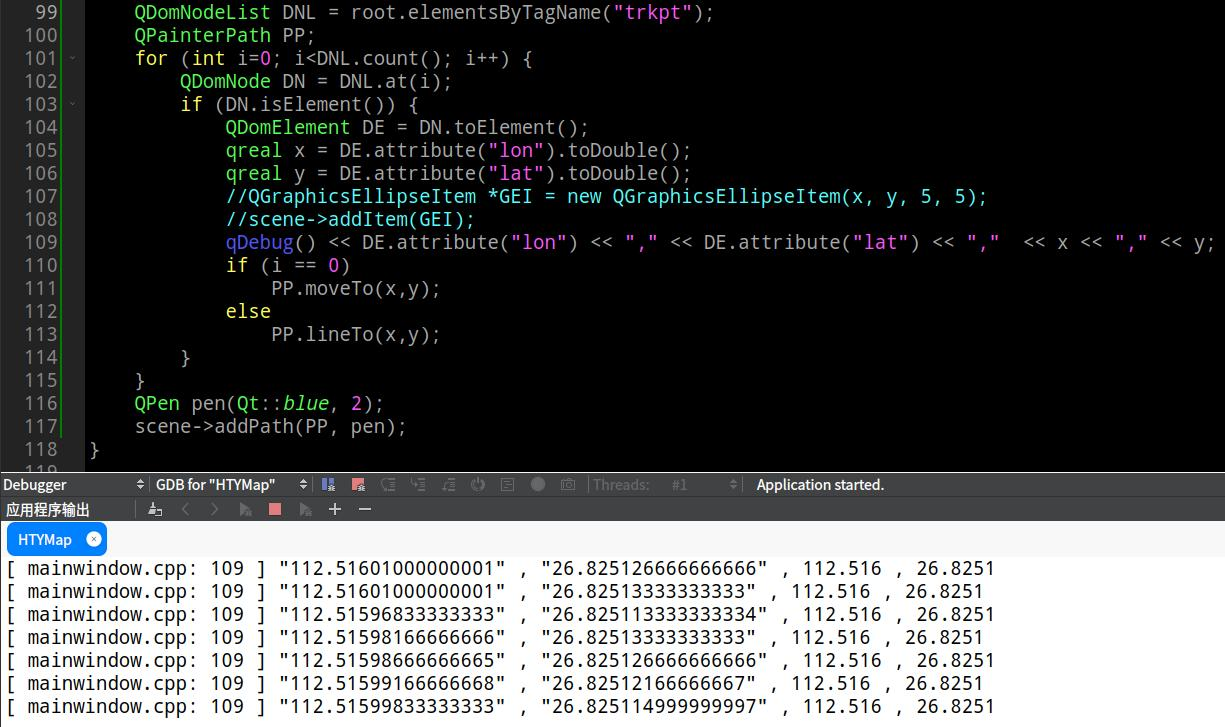# QString .toDouble() lose precision

• I am convert gpx file point to QPainterPath, when I use QString .toDouble() lose precision.

``````QFile file(filePath);
return;
QDomDocument doc;
if (!doc.setContent(&file)) {
file.close();
return;
}
file.close();
QDomElement root = doc.documentElement();
QDomNodeList DNL = root.elementsByTagName("trkpt");
QPainterPath PP;
for (int i=0; i<DNL.count(); i++) {
QDomNode DN = DNL.at(i);
if (DN.isElement()) {
QDomElement DE = DN.toElement();
qreal x = DE.attribute("lon").toDouble();
qreal y = DE.attribute("lat").toDouble();
qDebug() << DE.attribute("lon") << "," << DE.attribute("lat") << ","  << x << "," << y;
if (i == 0)
PP.moveTo(x,y);
else
PP.lineTo(x,y);
}
}
QPen pen(Qt::blue, 2);
``````• @sonichy
First, you should be aware that all C++ `double`s are approximate values. It cannot represent all the possible floating point values, only certain discrete ones. This is why, for example, you should not compare floating points values for equality with `==`, and Qt provides qFuzzyCompare.

Second, you are using `qDebug() << doubleValue`. Your output looks like that prints doubles with 6 significant digits. You will have to use a dedicated outputter with more significant digits to see more closely what the double value actually is.

• @JonB The QString.toDouble() is correct, the problem is the qDebug().

``````qDebug() << DE.attribute("lon") << "," << DE.attribute("lat") << ","  << QString("%1").arg(x, 0, 'f', 14) << "," << QString("%1").arg(y, 0, 'f', 14); //approximate
``````